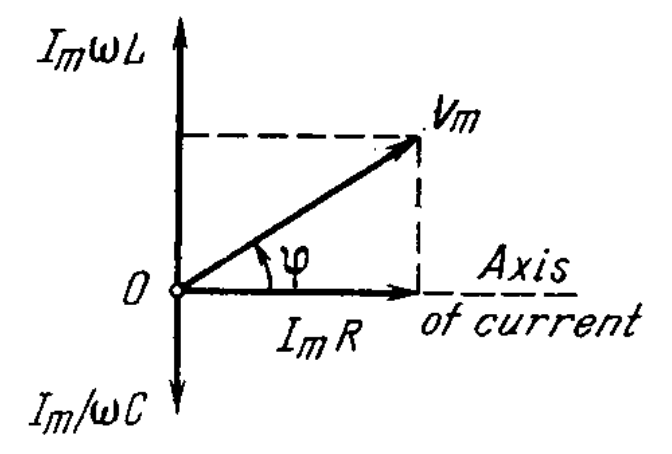# Electric oscillations

For page specific messages
For page author info

Damped oscillation in a circuit $$q=q_m e^{-\beta t} \cos(\omega t+ \alpha),$$ where $$\omega= \sqrt{ \omega ^2_0 -\beta^2, \omega_0=\frac{1}{\sqrt{LC}}}, \beta=\frac{R}{2L}.$$

• Damped oscillation in a circuit $$q=q_m e^{-\beta t} \cos(\omega t+ \alpha),$$ where $$\omega= \sqrt{ \omega ^2_0 -\beta^2}, \omega_0=\frac{1}{\sqrt{LC}}, \beta=\frac{R}{2L}.$$
• Logarithmic damping decrement $\lambda$ and quality factor $Q$ of a circuit are defined by Eqs.(4.1d). When damping is low:$$\lambda=\pi R\sqrt{\frac{C}{L}}, Q=\frac{1}{R}\sqrt{\frac{L}{C}}.$$
• Steady-state forced oscillation in a circuit with a voltage $V = V_m \cos \omega t$ connected in series:$$I=I_m \cos(\omega t - \phi),$$ where $$I_m=\frac{V_m}{\sqrt{ R^2 + (\omega L-\frac{1}{\omega C})^2}}, \tan \phi =\frac{\omega L- \frac{1}{\omega C}}{R}.$$ The corresponding vector diagram for voltages is shown in Fig. 4.26.• Power generated in an ac circuit:$$P=VI \cos \phi,$$ where $V$ and $I$ are the effective values of voltage and current:$$V=\frac{V_m}{\sqrt{2}}, I=\frac{I_m}{\sqrt{2}}.$$

y
0

X